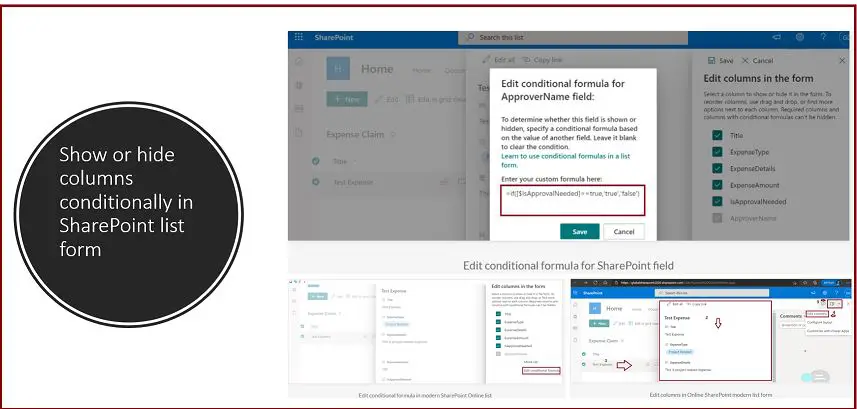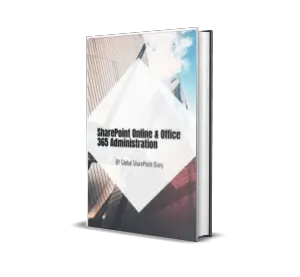# Conditionally show or hide columns in a SharePoint list

15,041 total views,  5 views today

In this conditionally show or hide columns in a SharePoint list article, we will learn how to show or hide columns conditionally in the SharePoint list form.

## Conditionally show or hide columns in a SharePoint list: what you will learn from this article?

• How to conditionally show and hide the column in SharePoint Online list form?
• How to conditionally show or hide columns conditionally in SharePoint list or library form?
• What are the supporting columns for the conditional formula in the SharePoint Online list or library?
• Unsupported column types in conditional formulas.
• How to work with logical operators in a conditional formula of SharePoint Columns?
• Conditional formula example with the allowed SharePoint column types.

In order to complete this demo, we need to have a Sharepoint list.

Here is the demo list, named “Expense Claim”

Below attached the list definition. Along with the “IsApproverNeeded(Yes/No type)” and “Approver Name (People/Group type)” – it has some other columns, however, here we are interested only in these two columns. The demo output would be as, if the user selects “IsApproverNeeded” then only the “Approver Name” column will be displayed otherwise would be hidden. Now, let’s get started with the demo.

We have the SharePoint Online list ready with sample data.

Click on the “Title” column hyperlink to open the list view form.

Then, the list item will be opened in the list view form.

Click on the “Edit Form (3)” dropdown list as shown in step 3.

Click on the “Edit columns” link.

Click on the three dots next to the column name for which you want to apply the formula. Here we have selected “ApproverName” as we want to hide and show this column based on the other column values changes (IsApproverNeeded – Yes/No).

Click on the “Edit conditional formula” link.

Enter the below text in the edit conditional formula box:

`=if([\$IsApprovalNeeded]==true,'true','false')`

Click on the “Save” button.

### Explanation about the above formula: conditionally show or hide column

• The above formula will return true if the “IsApproverNeeded” is selected as yes.
• Then the field “ApproverName” will be visible only when the formula returns true.

### Conditionally show or hide columns in a SharePoint list: what can be done using the conditional formula?

To determine whether this field is shown or hidden, specify a conditional formula based on the value of another field. Leave it blank to clear the condition.

## Show and hide column conditionally in SharePoint Online list – Demo:

Open the list item in edit mode.

We can notice that by default “IsApprovalNeeded” is unchecked, so we cannot see the “Approver Name” column – it is hidden.

Now, click on the “IsApprovalNeeded” checkbox, then we can see that the “Approver Name” column is being shown, and if we uncheck this selection

Note:

• If your SharePoint list column is a required one (Require that this column contains information:), this show and hide formula cannot be applied, as today this is the limitation from Microsoft.

Let’s check out the above limitation practically.

Make the “ApproverName” column as “Require that this column contains information” and click on the OK button.

Edit conditional formula in modern SharePoint Online list is hidden when the column is required:

Now, go again to the edit columns in the form configuration, and select the “ApproverName” column, we can notice that the “Edit conditional formula” is hidden now, only we can see the “Move up” option because we have made this column as a required.

### Working with various types of columns in SharePoint Online list for conditional formulas:

Type of the Column Formula Example
Number =if([\$Cost]>2000,’true’,’false’)
Choice =if([\$Department]==’HR’,’true’, ‘false’)
Date =if([\$ProjectStartDate]==Date(’17/03/2021′),’true’,’false’)
Person =if([\$ApproverFieldInternalName].email==’testuser@yourdomain.com’,’true’,’false’)
Yes/No (check box) =if([\$IsApproverNeeded]==true,’true’,’false’)

Note:

• We can use @me for the currently logged-in user for the filter.

We even can add/subtract the value of fields before comparing, below is the example:

`=if([\$Column1]+[\$Column2]<3000,'true','false')`

### Logical operators in the conditional formula in the SharePoint Online list

Logical operators are also allowed inside the conditional formula in SharePoint online list.

Logical Operator Description
&& Logical AND operator
|| Logical OR Operator
! Logical NOT Operator

## Different Types of Columns Example: Conditionally show or hide columns in a SharePoint list

### Choice column example:

`=if([\$ProjectCategory] == 'Full Time', 'true', 'false')`

### Number column example:

`=if([\$ProjectCost] <= 500, 'true', 'false')`

Even we can perform the addition of more than one column and compare it with the cost.

`=if(([\$ProjectCost] + [\$OtherCostcost]) > 1000, 'true', 'false')`

### Date column example:

`=if([\$ProjectStartDate] == Date('6/7/2021'), 'true', 'false')`

Even we can use the less than or equal to the condition in the date column:

=if([\$ProjectStartDate] <= Date(‘6/7/2021’), ‘true’, ‘false’)

### Two Date Columns combined with the && operators:

`=if([\$ProjectStartDate] >= Date('6/7/2021') && [\$ProjectEndDate] <= Date('6/10/2021'), 'true', 'false')`

### Person column example:

`=if([\$ProjectOwner.email] == 'ProjectOwner@global-sharepoint.com', 'true', 'false')`

### Boolean (Yes/No) column example:

`=if([\$IsProjectCostApproverRequired] == true, 'true', 'false')`

### Lookup Column: Conditionally show or hide columns in a SharePoint list

As of now, we have seen the example of generic column types in SharePoint online for the show and hide conditionally, now we will learn how to work with the Lookup column type, handling the lookup type is quite complicated than the other types of columns.

The lookup column condition can be constructed in two ways:

By checking the actual equal text string (value in the lookup column):

`=if(substring([\$YourLookUpColumn],indexOf([\$YourLookUpColumn],';#')+2,1000) == 'A value from the lookup column', 'true', 'false')`

Example:

`=if(substring([\$YourLookUpColumnCountry],indexOf([\$YourLookUpColumnCountry],';#')+2,1000) == 'India', 'true', 'false')`

By checking the index position of the text string (value in the lookup column):

`=if(Number(substring([\$YourLookUpColumn],0,indexOf([\$YourLookUpColumn],';#'))) == 1, 'true', 'false')`

Example:

`=if(Number(substring([\$YourLookUpColumnCountry],0,indexOf([\$YourLookUpColumnCountry],';#'))) == 1, 'true', 'false')`

Note:

• For the index example, assume that the country India is at the number 1 position in the lookup column value.

### Working with StartsWith functions in the Lookup column in show hide columns conditionally (JSON startswith)

Let’s say we have a project lookup column in a list where values are like – SharePoint Project1, SharePoint Project2, DotNet Project1, DotNet Project2, DotNet Project3, and so on.

Our requirement is whenever a user selects any of the SharePoint projects from the project lookup column, the “SharePoint Project Details” (another column in the same list type of Single Line of Text) column should be shown automatically otherwise it should be hidden. To achieve this, we can write the below formula with multiple OR conditions like below:

`=if(substring([\$ProjectLookUpColumn],indexOf([\$ProjectLookUpColumn],';#')+2,500) == 'SharePoint Project1' || substring([\$ProjectLookUpColumn],indexOf([\$ProjectLookUpColumn],';#')+2,500) == 'SharePoint Project2', 'true', 'false')`

This technique will work fine without any issues, however, let’s imagine you have 50 plus SharePoint projects, in this case, we need to write 50 OR conditions to check the SharePoint projects in the formula. This will be time-consuming to write the condition and maintenance also will not be easier. The same outcome we can achieve using just a single if condition with the “startsWith” function. Let’s see how does it work?

The below formula uses the “startsWith” function, which gives the same output as the above formula which has multiple conditions.

`=if(startsWith(substring([\$ProjectLookUpColumn],indexOf([\$ProjectLookUpColumn],';#')+2,500),'SharePoint'), 'true', 'false')`

Explanation:

• This formula uses the “startsWith” function which checks – if any values from the “ProjectLookUpColumn” column start with the text “SharePoint”, for those the “SharePoint Project Details” (another column in the same list type of Single Line of Text) column will be visible otherwise it will be hidden. Here we have just used a single if condition.

### How to handle an empty and not empty condition in conditionally show or hide columns in a SharePoint list

Checking empty conditions in JSON conditional formatting is completely different, it is not like “!=” not equal  or “==” ”. For example, if your project status column is not empty, then you want to hide a column (a single line text column – it could be any above-mentioned columns). Then the formula would be as below:

`=if([\$ProjectStatus], 'false', 'true')`

Reverse ways, if your project status column is empty, then you want to hide a column (a single line text column – it could be any above-mentioned columns). Then the formula would be as below:

`=if([\$ProjectStatus], 'true', 'false')`

Note:

• The above formula should be written in the column that you want to show or hide conditionally based on other column values. Here based on the project status column value “YourSingleLineTextColumn” will be hidden or shown.
• The above empty or not empty conditional show hide column is needed in many cases – for example, for the calculation purpose, or for Power Automate trigger point, we might need some hidden column which should not be displayed in the form but we can use that column for to handling the business logic.

## Limitation – column types not supported in conditional formulas in SharePoint list/library: Unsupported column types in conditional formulas

As of today, the below list of columns is not supported in the conditional formula in the SharePoint list.

• Choice with multiple selections
• Time calculations in the Date and Time column
• Currency columns
• Location columns
• Calculated columns
• Person or Group with multiple selections

## A few points must remember: Conditionally show or hide columns in a SharePoint list

• Column names are always internal names – display names will not work.
• The JSON conditional formula will not work in the Quick Edit (Grid View) view, this is the limitation we must know and let your client knows about it upfront. For this, if the client agrees, we can disable the Quick Edit (Grid View) view for that list (by going to the list advanced settings options, set the  “Quick property editing” to  No, by default this will be Yes).
• The value in the condition is case sensitive – for example instead of “true” and “false” if you write “True” and “False” will not work.

## Summary: Conditionally show or hide columns in a SharePoint list

Thus, in this article we have learned the below with respect to showing and hiding columns conditionally in SharePoint Online list form:

• How to conditionally show and hide the column in SharePoint Online list form?
• How to conditionally show or hide columns conditionally in SharePoint list or library form?
• What are the supporting columns for the conditional formula in the SharePoint Online list or library?
• Unsupported column types in conditional formulas.
• How to work with logical operators in a conditional formula of SharePoint Columns?
• Conditional formula example with the allowed SharePoint column types (Single Line Text, Choice, Yes/No, People, Number, Date)
• Working with StartsWith functions in the Lookup column in show hide columns conditionally.

## Article Recap: Conditionally show or hide columns in a SharePoint listConditionally show or hide columns ...Please enable JavaScriptConditionally show or hide columns in a SharePoint Online